# Anyone Can Learn Anything

## Let’s draw a cool plot 2

Let us start from the point where we stopped in the last lecture. There are many things we can do for making our plot looks cooler. One of the main things we should consider is a getting a title and name of axes of our plot!

We can labeling of our plot by setting main, xlab, and ylab arguments in the plot function as follows:

x <- seq(-10, 10, length.out = 100)
y <- x^2
plot(x,y,
main = "Plot of y = x^2",    # make title
xlab = "x axis",             # x axis labelling
ylab = "y axis",             # x axis labelling
xlim = c(-2,2),              # range of x axis
ylim = c(0,4),               # range of y axis
type = 'l',                  # choose line type
col = "Blue"                 # choose line color
)
abline(h = 0:4, v = -2:2, col = "Gray", lty=3)
abline( v = 0, lty = 1)           # adding y axis
abline( h = 0, lty = 1)           # adding x axis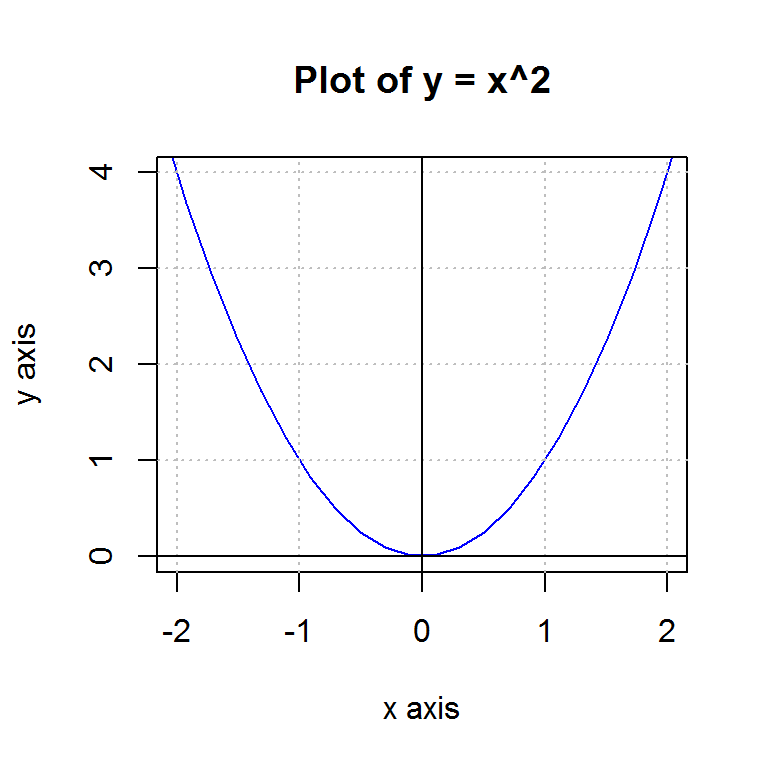We have a little problem here. Now we have a title with expression “x^2” but this is not what we want as a title. By using a function expression(), we can use a mathematical title. Let us change our code as follows:

plot(...
main = expression(paste("Plot of ", y, " = ", x^2)),
...
)

Then, we will have a plot as follows: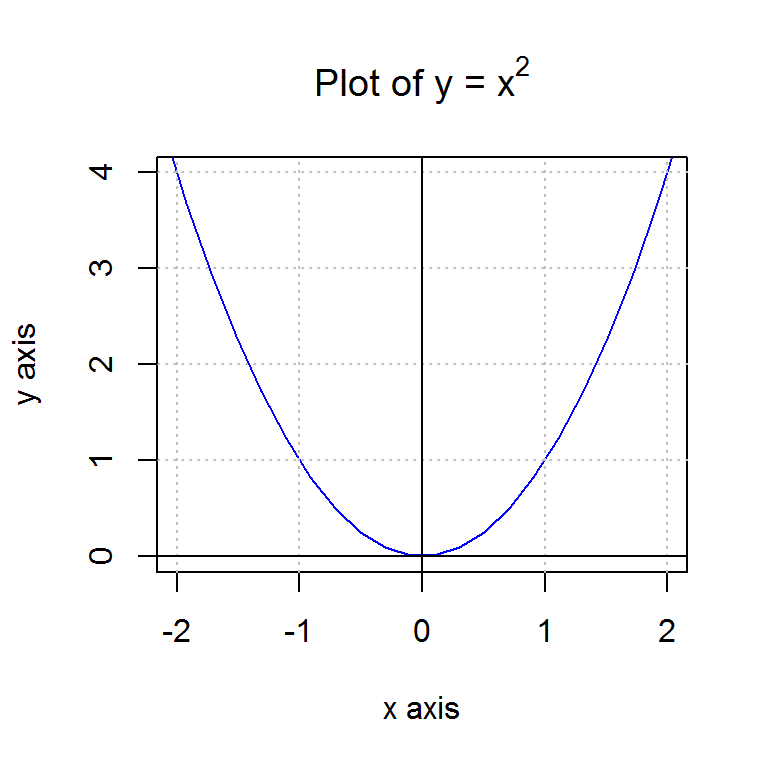In the code, paste() function is used for manipulating the text. This function allows the user to ‘literally’ paste multiple texts into one. Here is a toy example:

paste("a", "and", "b")
##  "a and b"

We will study this function again in the text manipulation posting.

Now, our plot looks fancy when we generated the first time. But, it looks a little bit boring. We need color! Let us try to change the background color so that our plot looks more attractive. Note that, from now on, the code might be tricky to some people but if you try to follow my explanation step by step, you finally get it!

Let me show you where we are heading to first. This is the result and R code that we will finally get it.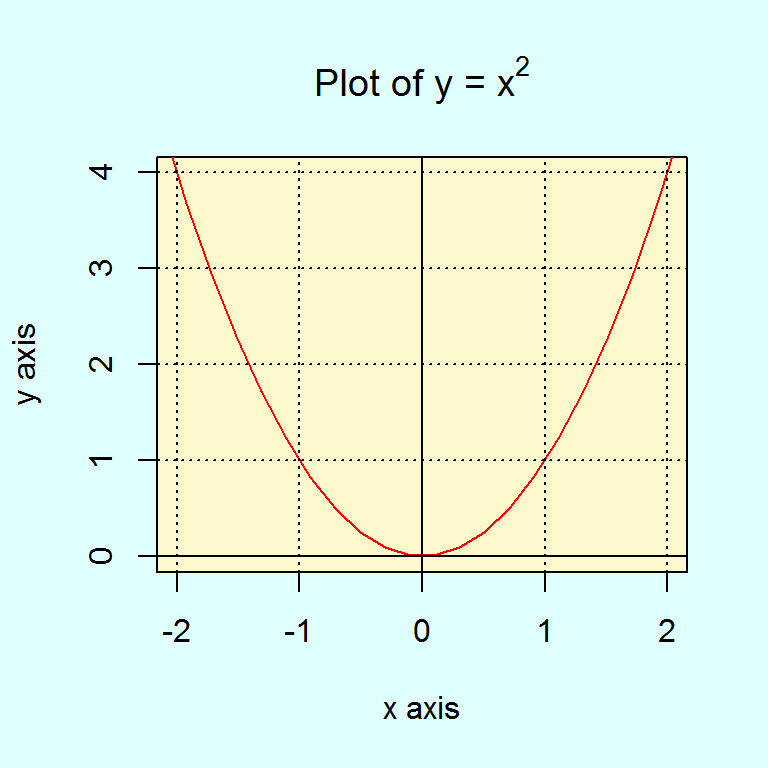par(bg = "light cyan")  # set the color of pallet

# Generate empty plot
plot(c(-2,2),c(0,4),    # just two points
main = expression(paste("Plot of ", y, " = ", x^2)),
xlab = "x axis",
ylab = "y axis",
xlim = c(-2,2),
ylim = c(0,4),
type = 'n',        # line type is null!
col = "Blue")

# Draw gray rectangle
loc <- par("usr")
rect(loc, loc, loc, loc,
col = "lemonchiffon")

# Draw a red line
points(x,y, type = 'l', col = "Red")

# Setting reference lines
abline(h = 0:4, v = -2:2, col = "black", lty=3)
abline( v = 0, lty = 1)
abline( h = 0, lty = 1)

Well, looks complicated but it’s not. First, we need to understand the concept of ‘pallet’. So far, we didn’t pay attention to the pallet concept since we only need to draw one plot. When we want to draw multiple plots, we need to set the layout of the pallet. Since we will study how to draw multiple plots later, now I will tell you what the pallet is.

Let us write the command par() in the console and execute it. This code will change the color of what it called ‘pallet’.

par(bg = "Yellow")

It looks nothing happen. However, when you enter plot(c(0,1),c(0,1)) command, you will see what has been changed.

par(bg = "Yellow")
plot(c(0,1),c(0,1))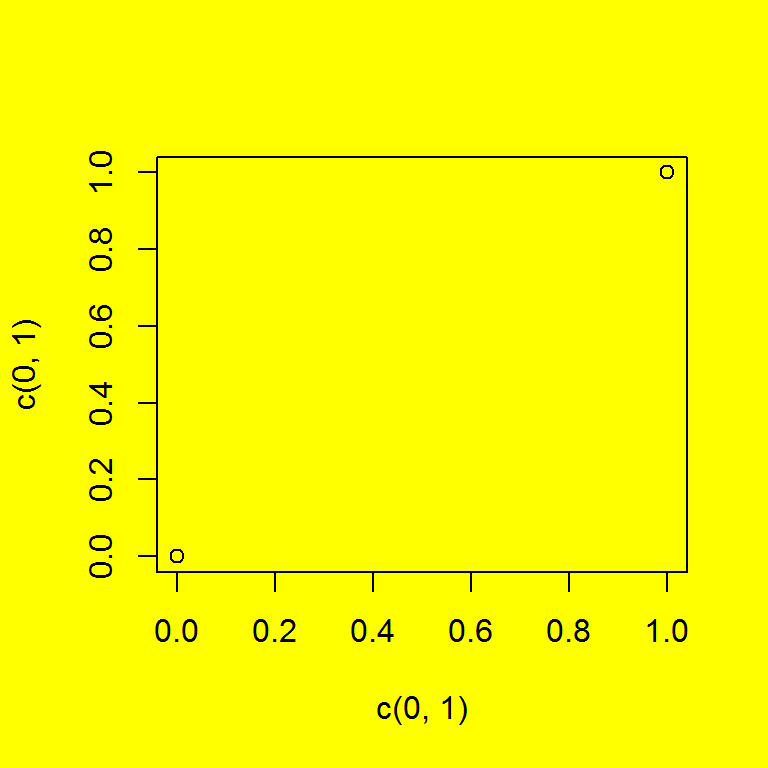Thus, we draw a plot on the yellow box called pallet, whose color was white before we changed. On this pallet, we draw a plot of two points; (0,0) and (1,1). If you use option type = ‘n’ in given code, R returns an empty plot. Next, what we are going to do is that we will draw a rectangle which fill the square area. The rect() function allows us to draw a rectangle. The syntax of the rect() is as follows:

rect(xleft, ybottom, xright, ytop, density = NULL, angle = 45,
col = NA, border = NULL, lty = par("lty"), lwd = par("lwd"),
...)

Thus, it seems that we need to fill the area from 0 to 1 with respect to x and y axes. However, let us see what happen we draw a rectangle as follows:

par(bg = "Yellow")
plot(c(0,1),c(0,1))
loc <- par("usr")
rect(0,0,1,1, col = "blue")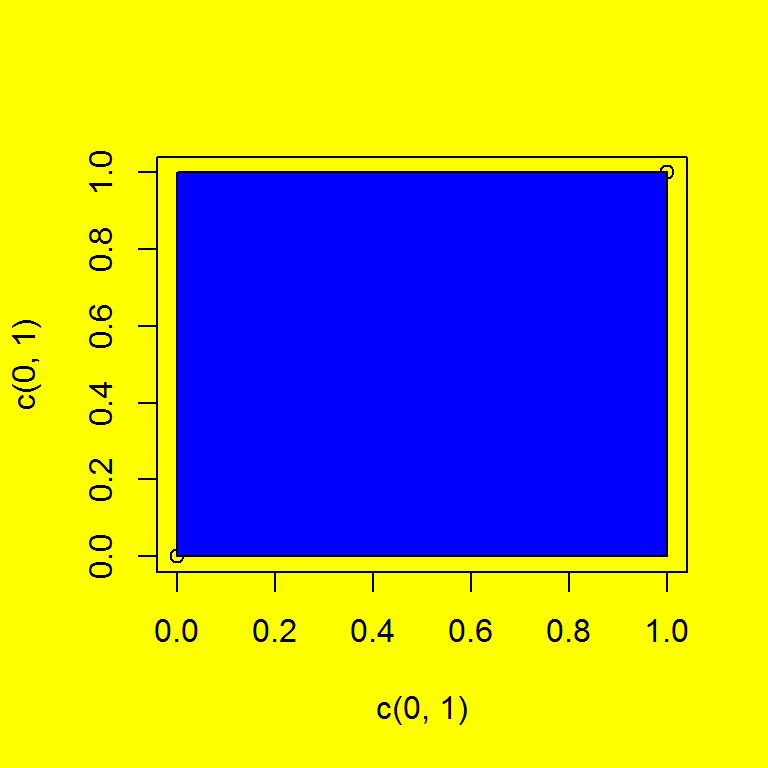As we can see, to fill the entire area of the square, we need the bigger one. However, the problem is that we don’t know the exact coordinate of the each edge of the square. These points are contained in par(“usr”) object. Since we assign the values of par(“usr”) to the variable loc, let us check the which values are in the variable loc.

loc
##  -0.04  1.04 -0.04  1.04

loc variable contains the coordinates of the edge of the square: left x value, right x value, bottom y value, top y value respectively. Thus, there are 0.04 margins between rectangle we draw and the square area that needs to be filled. Using this fact, we can fill the square as follows:

par(bg = "light cyan")
plot(c(0,1),c(0,1))
loc <- par("usr")
rect(loc, loc, loc, loc,
col = "lemonchiffon")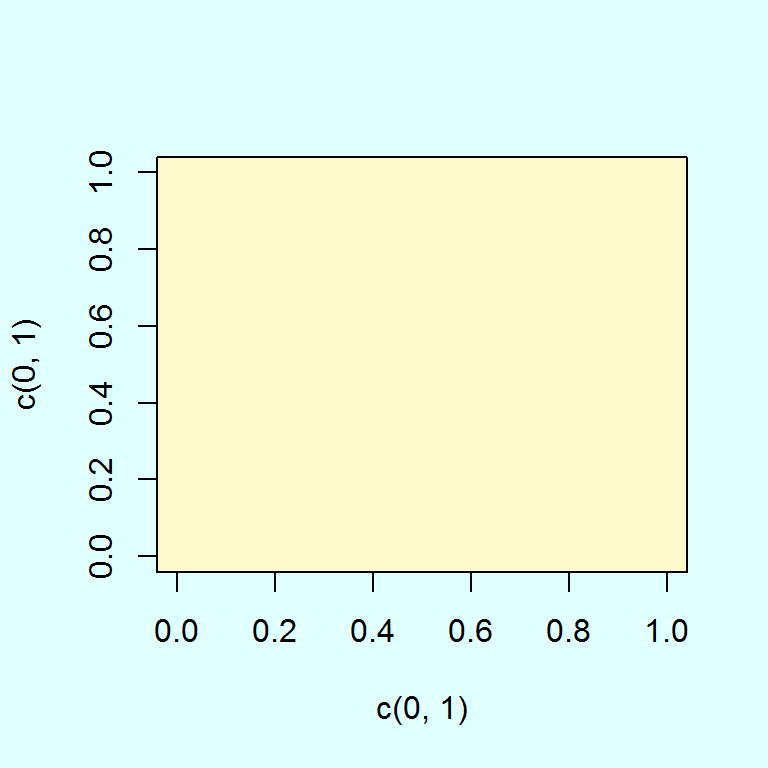Now, we are almost done. The only thing we left is learning how to add some points in the given plot. Since we have already plot object, we cannot use plot() anymore. Instead of doing that, we can add points to the plot connected with lines. The following R code will add two red points at (0,0) and (1,1).

par(bg = "light cyan")
plot(c(0,1),c(0,1))
loc <- par("usr")
rect(loc, loc, loc, loc,
col = "lemonchiffon")
points(c(0,1),c(0,1), type = 'p', col = "Red")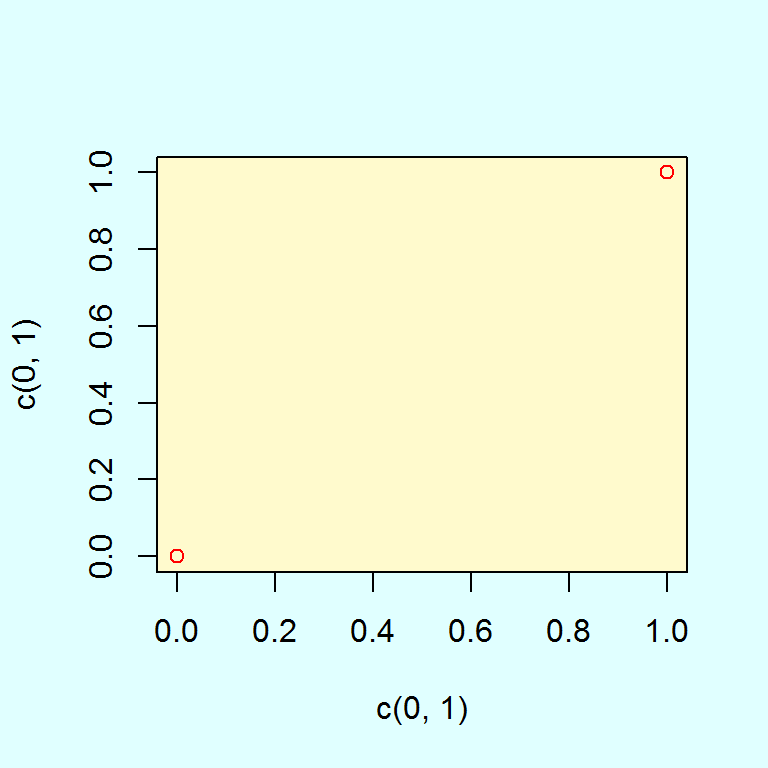Now, if you understand all things we have learned, you definitely can see what was going on the previously given code. Hope you can understand every single line!

par(bg = "light cyan")  # set the color of pallet

# Generate empty plot
plot(c(-2,2),c(0,4),    # just two points
main = expression(paste("Plot of ", y, " = ", x^2)),
xlab = "x axis",
ylab = "y axis",
xlim = c(-2,2),
ylim = c(0,4),
type = 'n',        # line type is null!
col = "Blue")

# Draw gray rectangle
loc <- par("usr")
rect(loc, loc, loc, loc,
col = "lemonchiffon")

# Draw a red line
points(x,y, type = 'l', col = "Red")

# Setting reference lines
abline(h = 0:4, v = -2:2, col = "black", lty=3)
abline( v = 0, lty = 1)
abline( h = 0, lty = 1)Past posting

2017/01/11 - [Programming/R] - Let's draw a cool plot 1

2017/01/09 - [Programming/R] - Vector filtration in R

2017/01/09 - [Programming/R] - Vector declaration in R 2

#### 'R Programming > Graphics' 카테고리의 다른 글

 ggplot2 패키지 정리 - 1. stat_density, stat_function, geom_rug, geom_point, anotate, labs  (0) 2017.07.11 2017.05.28 2017.05.28 2017.05.28 2017.05.28 2017.05.28 2017.05.24 2017.05.24 2017.01.11 2017.01.10